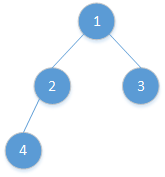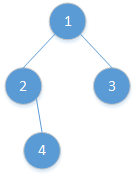# 题目描述## 思路分析

### 递归

1. 当前节点为null时，return "";
2. 左右节点均无：return String.valueOf(root.val);
3. 只存在左节点：return root.val + "(" + solution(root.left) + ")";
4. 只存在右节点： return root.val + "()(" + solution(root.left) + ")";
5. 左右节点均存在：return root.val + "(" + solution(root.left) + ")(" + solution(root.right) + ")";

        if (root == null) {
return "";
}

if(root.right == null){
if(root.left == null)
return String.valueOf(root.val);
else
return root.val
+ "("
+ solutionRecursive(root.left)
+ ")";
}

// root.left != null && root.right != null
return root.val
+ "("
+ solutionRecursive(root.left)
+ ")("
+ solutionRecursive(root.right)
+ ")";


### 迭代

Stack<TreeNode> stack = new Stack<>();
HashSet<TreeNode> visitedSet = new HashSet<>();
StringBuilder res = new StringBuiler();

stack.push(root);

while(!stack.empty()){
TreeNode node = stack.peek();
if(visitedSet.contains(node)){
stack.pop();
res.append(")");
}else{
visitedSet.add(node);
res.append("(" + node.val);

if(node.left == null && node.right != null)
res.append("()");

if(node.right != null) // attention here !!!!
stack.push(node.right);
if(node.left!= null)
stack.push(node.left);
}
}

return res.subString(1, res.length() - 1);


## 解题代码

### 1. 递归

    public static String solutionRecursive(TreeNode root) {
if (root == null) {
return "";
}

if(root.right == null){
if(root.left == null)
return String.valueOf(root.val);
else
return root.val
+ "("
+ solutionRecursive(root.left)
+ ")";
}

// root.left != null && root.right != null
return root.val
+ "("
+ solutionRecursive(root.left)
+ ")("
+ solutionRecursive(root.right)
+ ")";

}


### 2. 迭代

    public static String solutionIterative(TreeNode root){
if(root == null) return "";

StringBuilder res = new StringBuilder();
HashSet<TreeNode> visitedSet = new HashSet<>();
Stack<TreeNode> stack = new Stack<>();

stack.push(root);

while(!stack.empty()){
TreeNode node = stack.peek();
if(visitedSet.contains(node)){
res.append(")");
stack.pop();
}else{
visitedSet.add(node);
res.append("(" + node.val);

if(node.left == null && node.right != null)
res.append("()");
if(node.right != null)
stack.push(node.right);
if(node.left != null)
stack.push(node.left);

}
}
return res.substring(1, res.length() - 1);

}


## 复杂度分析

1. 递归

1. 迭代
时间复杂度： 时间复杂度为O(n);
空间复杂度： 我们这里借助了stack, visited，以及返回结果res，最坏情况下为3n，故空间复杂度为O(n);

## Github源码

05-14711
05-2320208-1333
12-2665万+
03-2199
07-022062
12-1782
11-24104
08-22914
01-2292
04-12286
07-27256
12-0953
11-07816
10-30146
02-234136
05-218439
12-1312
04-102万+
©️2020 CSDN 皮肤主题: 程序猿惹谁了 设计师:白松林